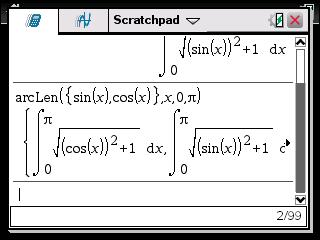# Knowledge Base

## Solution 33551: Finding the Arc Length of an Expression on the TI-Nspire™ CAS Family Line.

### How do I find the arc length on the TI-Nspire CAS family line?

To find the arc length fo an expression from a start to an end use the arcLen() function. The arcLen() function returns the arc length of an expression from start to end with respect to the variable var. When used with a list, it returns a list of arc lengths of each element of the list from start to end, with respect to var. Regardless of the graphing mode, arc length is calculated as a integral assuming a function mode definition. The syntax of the arcLen() function is as follows:

arcLen(expression1,var,start,end)
arcLen(list1,var,start,end)

Example:

Find the arc length of cosine(X) from 0 to π.

• Press [home] [A].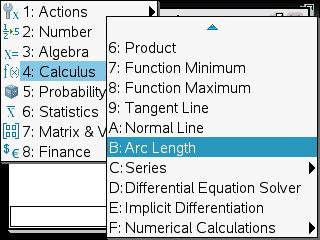• Press [trig►] [►] [enter].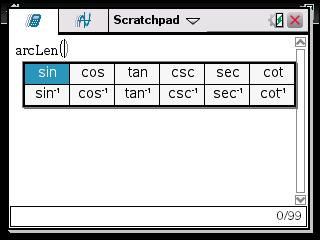• Press [X] [►] [X] [,]  [,]
• Press [π►] [enter].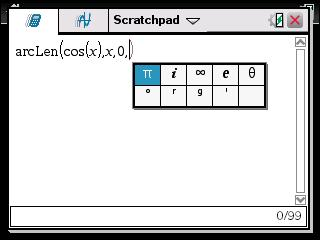• Press [enter].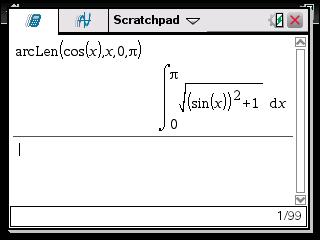Second example:

Find the arc length of list {Sine(X), Cosine(X)} from 0 to π.